# The Eady model for baroclinic instability¶

## Rotating reference frame¶

### The Coriolis term and the "traditional approximation"¶

In a rotating frame of reference there is an extra term that appears in the momentum equations: the Coriolis term \begin{align*} 2\boldsymbol{\Omega}\boldsymbol{\times}\boldsymbol{u}. \tag{1} \end{align*} Consider the expression of the Coriolis force in cartesian coordinates where $x$ is along latitude circles, $y$ along longitudes, and $z$ is in the vertical. Then,

\begin{align*} \boldsymbol{\Omega}\boldsymbol{\times}\boldsymbol{u} &= \left|\begin{array}{ccc}\widehat{\boldsymbol{x}}&\widehat{\boldsymbol{y}}&\widehat{\boldsymbol{z}}\\0 &\Omega\cos{\theta}&\Omega\sin{\theta}\\ u & \upsilon& w\end{array}\right| \\&= \big( 2\Omega w \cos\theta - 2\Omega \upsilon\sin\theta\big)\widehat{\boldsymbol{x}} + 2\Omega u\sin\theta\,\widehat{\boldsymbol{y}} - 2\Omega u \cos\theta\,\widehat{\boldsymbol{z}}. \tag{2} \end{align*}

### The Coriolis term and the "traditional approximation"¶

A usual approximation is the so called "traditional approximation", in which terms involving the horizontal component of the Earth’s angular velocity are neglected. With these approximations we have that

\begin{align*} 2\boldsymbol{\Omega}\boldsymbol{\times}\boldsymbol{u} \approx - 2\Omega \upsilon\sin\theta\,\widehat{\boldsymbol{x}} + 2\Omega u\sin\theta\,\widehat{\boldsymbol{y}}, \tag{3} \end{align*}

and the equations of motions simplify to:

\begin{align*} (\partial_t + \boldsymbol{u}\boldsymbol{\cdot}\boldsymbol{\nabla})\,u - f \upsilon &= -\partial_x p\big/\rho_m, \tag{4} \\ (\partial_t + \boldsymbol{u}\boldsymbol{\cdot}\boldsymbol{\nabla})\,\upsilon + f u &= -\partial_y p\big/\rho_m, \tag{5}\\ (\partial_t + \boldsymbol{u}\boldsymbol{\cdot}\boldsymbol{\nabla})\,w &= -\partial_z p\big/\rho_m + b, \tag{6}\\ (\partial_t + \boldsymbol{u}\boldsymbol{\cdot}\boldsymbol{\nabla})\,b &= 0, \tag{7} \end{align*}

where we have defined $f\equiv 2\Omega\sin\theta$, where $\theta$ is the latitude.

[There are some intermediate steps still to get the quasi-geostrophic (QG) equations...]

### Quasi-geostrophic (QG) equations¶

In QG approximation the vertical velocity is zero (at leading order) and the horizonal velocities $u,\ \upsilon$ are given in terms of a streamfunction: $u=-\partial_y\psi$ and $\upsilon=\partial_x\psi$. The streamfunction is proportional to the pressure: $\psi=p\big/(\rho_m f_0)$. Furthermore, from hydrostatic balance, we have that buoyancy is also expressed in terms of the streamfunction $b=f_0\partial_z\psi$.

After some fiddling around we get that the equations of motion are:

\begin{align*} (\partial_t + u\partial_x + \upsilon\partial_y)\,q &= 0\quad\text{for }0<z/H<1, \tag{8}\\ (\partial_t + u\partial_x + \upsilon\partial_y)\,b &= 0\quad\text{@ }z=0, H, \tag{9} \end{align*}

where $q = f_0 + \partial_x\upsilon - \partial_y u + \partial_z\big(\frac{f_0}{N^2(z)}b\big)$ is the quasi-geostrophic potential vorticity (QGPV) and $b$ is the buoyancy. The QG approximation is very good for atmospheric flows in the mid-latitude. In that case, $z=0$ corresponds to the Earth's surface and $z=H$ corresponds to the tropopause, which is at about 10 km in the mid-latitudes.

In terms of the streamfunction the QGPV and buoyancy are:

\begin{align*} q = f_0 + \big(\partial_x^2+\partial_y^2 + \partial_z \frac{f_0^2}{N^2} \partial_z\big)\psi \quad\text{and}\quad b=f_0\partial_z\psi. \tag{10} \end{align*}

The domain-averaged energy of the flow is

\begin{align*} E &= \frac1{H L_x L_y}\int \frac1{2}\big( u^2 + \upsilon^2 + \frac{b^2}{N^2}\big)\,\mathrm{d}^3\boldsymbol{x}\\ &= \frac1{H L_x L_y}\int \frac1{2}\big[ (\partial_x\psi)^2 + (\partial_y\psi)^2 + \frac{f_0^2}{N^2} (\partial_z\psi)^2\big]\,\mathrm{d}^3\boldsymbol{x}. \tag{11} \end{align*}

Also, since everything is given in terms of $\psi$ it's also obvious that the following hold:

\begin{align*} f_0\partial_z u = -\partial_y b \quad\text{and}\quad f_0\partial_z \upsilon = \partial_x b. \tag{12} \end{align*}

These are called "thermal wind relations" and they relate the vertical gradients of the flow with horizontal buoyancy gradients.

Let's consider a constant stratification, $N^2(z)=\textrm{const}$. (This is equivalent with $\mathrm{d}\rho_0/\mathrm{d}z=\textrm{const}$.) In this case we can see that the streamfunction

\begin{align*} \psi^e = -\Lambda y z,\tag{13} \end{align*}

satisfies the QG equations. This streamfunction gives:

\begin{align*} u^e = \Lambda z,\ \upsilon^e=0,\ q^e = 0,\ b^e=-f_0\Lambda y.\tag{14} \end{align*}

We want to study how perturbations about solution (13) evolve. For simplicity, we will take perturbations that are independent of $y$, i.e., $\psi=\psi^e+\psi'(x,z,t)$. The linearized QG equations about the basic state become:

\begin{align*} \big(\partial_t + u^e_+\partial_x \big)b'_+ + \upsilon'_+\partial_yb^e_+ &= 0\quad\text{@ }z=H,\tag{15}\\ \big(\partial_t + u^e\partial_x \big)q' &= 0\quad\text{for }0\le z/H\le 1,\tag{16}\\ \big(\partial_t + u^e_-\partial_x \big)b'_- + \upsilon'_-\partial_yb^e_- &= 0\quad\text{@ }z=0,\tag{17} \end{align*}

with subscripts $-/+$ denoting the values of the fiends at $z=0$ and $z=H$ respectively. Expressed in terms of streamfuction we have:

\begin{align*} \big(\partial_t + \Lambda H\partial_x \big)\partial_z\psi'_+ - \Lambda\partial_x\psi'_+ &= 0\quad\text{@ }z=H,\tag{18}\\ \big(\partial_t + \Lambda z\partial_x \big)\big(\partial_x^2 + \frac{f_0^2}{N^2}\partial_z^2\big)\psi' &= 0\quad\text{for }0\le z/H\le 1,\tag{19}\\ \partial_t \partial_z\psi'_- - \Lambda\partial_x\psi'_- &= 0\quad\text{@ }z=0,\tag{20} \end{align*}

### Non-dimensionalization¶

It's always very important to try to reduce the number of free parameters by non-dimensionalizing appropriately. Finding the best way to non-dimensionalize a system is a bit of an art. In this case, after fiddling around we get that if we use:

\begin{align*} z = H\widetilde{z},\ x = \frac{NH}{f_0}\widetilde{x},\ t=\frac{N}{\Lambda f_0}\widetilde{t}.\tag{21} \end{align*}

where tildes denote non-dimensional variables. Furthermore, we can scale the streamfunction as

\begin{align*} \psi' = \frac{NH^2\Lambda}{f_0} \widetilde{\psi}'.\tag{22} \end{align*}

However, because our equations are linear in perturbation streamfunction, how we scale $\psi'$ does not really matter...

Putting all these together, the linearized equations of motions become:

\begin{align*} \big(\partial_{\widetilde{t}} + \partial_{\widetilde{x}} \big)\partial_{\widetilde{z}} \widetilde{\psi}'_+ - \partial_{\widetilde{x}} \widetilde{\psi}'_+ &= 0\quad\text{@ }\widetilde{z}=1,\tag{23}\\ \big(\partial_{\widetilde{t}} + \widetilde{z} \partial_{\widetilde{x}} \big)\big(\partial_{\widetilde{x}}^2 + \partial_{\widetilde{z}}^2\big)\widetilde{\psi}' &= 0\quad\text{for }0\le \widetilde{z}\le 1,\tag{24}\\ \partial_{\widetilde{t}} \partial_{\widetilde{z}}\widetilde{\psi}'_- - \partial_{\widetilde{x}} \widetilde{\psi}'_- &= 0\quad\text{@ }\widetilde{z}=0.\tag{25} \end{align*}

Remarkably most free parameters are gone! Only the zonal wavenumber remains. Now we are ready to code-up these equations.

In non-dimensional variables the energy of the perturbations is

\begin{align*} E &= \frac1{H L_x}\int \frac1{2}\big[ (\partial_x\psi)^2 + \frac{f_0^2}{N^2} (\partial_z\psi)^2\big]\,\mathrm{d}^2\boldsymbol{x} \\ &= \frac{N H^3 \Lambda^2}{f_0L_x}\int \frac1{2}\big[ (\partial_{\widetilde{x}}\psi)^2 + (\partial_{\widetilde{z}}\psi)^2\big]\,\mathrm{d}\widetilde{x}\,\mathrm{d}\widetilde{z}. \tag{26} \end{align*}

From here after we will use the nondimensional equations but dropping the tildes.

### Homogeneity in $x$¶

Given that the equations are homogeneous in $x$ we can take

\begin{align*} \psi'(x,z,t)= \widehat{\psi}(z,t)e^{\mathrm{i}kx}.\tag{27} \end{align*}

which implies:

\begin{align*} (\partial_t +\mathrm{i}k)\partial_z\widehat{\psi}_+ - \mathrm{i}k \widehat{\psi}_+ &= 0\quad\text{@ }z=1,\tag{28}\\ (\partial_t + \mathrm{i}k z )(\partial_z^2-k^2)\widehat{\psi} &= 0\quad\text{for }0\le z\le 1,\tag{29}\\ \partial_t \partial_z\widehat{\psi}_- - \mathrm{i}k\widehat{\psi}_- &= 0\quad\text{@ }z=0.\tag{30} \end{align*}

If we compute the energy averaged over a zonal wavelength (i.e., $L_x\to 2\pi/k$) and use our expansion (27) we get:

\begin{align*} E &=\frac{H^2 \Lambda^2}{4} \int \big( |\partial_z\widehat{\psi}|^2 + k^2 |\widehat{\psi} |^2 \big)\,\mathrm{d}\widetilde{z}.\tag{31} \end{align*}

### Numerical implementation¶

Assume a discretization of our $z$-domain as:

\begin{align*} z \to \underbrace{z_1}_{=0}, z_2, \dots, z_{n_z-1}, \underbrace{z_{n_z}}_{=1}, \tag{32} \end{align*}

and similarly

\begin{align*} \widehat{\psi}(z, t) \to \underbrace{{\psi}_1}_{=\widehat{\psi}(0,t)}, {\psi}_2, \dots, {\psi}_{n_z-1}, \underbrace{\widehat{\psi}_{n_z}}_{=\widehat{\psi}(z_{n_z},t)}.\tag{33} \end{align*}

The QGPV equations become

\begin{align*} \partial_{t}\big(\frac{\psi_{j+1}-2\psi_j+\psi_{j-1}}{\delta^2}-k^2\psi_j\big) &= -\mathrm{i} k z_j\big(\frac{\psi_{j+1}-2\psi_j+\psi_{j-1}}{\delta^2}-k^2\psi_j\big)\quad\text{for }j=1,\dots,n_z.\tag{34} \end{align*}

But this means the QGPV equations at the boundaries require $\psi_{-1}$ and $\psi_{n_z+1}$ which are outside of our domain! However we can get around that using the extra two buoyancy equations at the boundaries. For example, the buoyancy equation at $z=0$ implies

\begin{align*} \partial_{t} \frac{\psi_2-\psi_{0}}{2\delta}& = \mathrm{i}k \psi_1.\tag{35} \end{align*}

We can eliminate $\psi_{0}$ by multiplying (35) with $2/\delta$ and add to it equation (34) for $j=1$. We then have:

\begin{align*} \partial_{t}\big(\frac{2\psi_{2}-2\psi_1}{\delta^2}-k^2\psi_1\big) &= \frac{2\mathrm{i}k}{\delta}\psi_1. \tag{36} \end{align*}

Similarly, we can eliminate $\psi_{n_z+1}$ by using the buoyancy equation at $z=1$. That gives:

\begin{align*} \partial_{t}\big(\frac{-2\psi_{n_z}+2\psi_{n_z-1}}{\delta^2}-k^2\psi_{n_z}\big) &= -\mathrm{i}k \big(\frac{-2\psi_{n_z}+2\psi_{n_z-1}}{\delta^2}-k^2\psi_{n_z}\big) - \frac{2\mathrm{i}k}{\delta}\psi_{n_z}. \tag{37} \end{align*}

Thus our equations are (36), (37) and

\begin{align*} \partial_{t}\big(\frac{\psi_{j+1}-2\psi_j+\psi_{j-1}}{\delta^2}-k^2\psi_j\big) &= -\mathrm{i} k z_j\big(\frac{\psi_{j+1}-2\psi_j+\psi_{j-1}}{\delta^2}-k^2\psi_j\big)\quad\text{for }j=2,\dots,n_z-1. \tag{38} \end{align*}

We have $n_z+1$ equations for $n_z+1$ variables. (It always feels good when one realises that.)

Next we want to express the energy integral in discrete variables. For the potential energy term we have:

\begin{align*} \int |\partial_z\widehat{\psi}|^2 \,\mathrm{d}\widetilde{z} &\to \frac1{2} \big[\partial_z\widehat{\psi}^*\big]_1\big[\partial_z\widehat{\psi}\big]_1 \delta + \big[\partial_z\widehat{\psi}^*\big]_2\big[\partial_z\widehat{\psi}\big]_2 \delta + \dotsb +\big[\partial_z\widehat{\psi}^*\big]_{n_z-1}\big[\partial_z\widehat{\psi}\big]_{n_z-1} \delta + \frac1{2}\big[\partial_z\widehat{\psi}^*\big]_{n_z}\big[\partial_z\widehat{\psi}\big]_{n_z} \delta.\tag{39} \end{align*}

We'll approximate the derivatives at the boundaries as, e.g.,

while for the interior terms we will use the average of the forward and backwards finite difference, e.g.,

\begin{align*} \big[\partial_z\widehat{\psi}^*\big]_j\big[\partial_z\widehat{\psi}\big]_j = \frac1{2}\Big(\frac{\psi_{j+1}^*-\psi_j^*}{\delta}\frac{\psi_{j+1}-\psi_j}{\delta} + \frac{\psi_{j}^*-\psi_{j-1}^*}{\delta}\frac{\psi_{j}-\psi_{j-1}}{\delta}\Big).\tag{41} \end{align*}

Therefore we have that

\begin{align*} \frac{E}{2\pi/k} = \int |\partial_z\widehat{\psi}|^2 + k^2|\psi|^2 \,\mathrm{d}\widetilde{z} &\to \big[ \psi_1^*,\dots,\psi^*_{n_z}\big]\,\mathbb{M}\begin{bmatrix} \psi_1\\\vdots\\\psi_{n_z}\end{bmatrix},\tag{42} \end{align*}

with

\begin{align*} \mathbb{M} \equiv \frac1{\delta} \begin{pmatrix} 1+\frac1{2}k^2\delta^2 & -1 & 0 & \dotsb & 0 \\ -1 & 2+k^2\delta^2 & -1 & & \vdots \\ 0 & \ddots & \ddots & \ddots & 0\\ \vdots & & -1 & 2+k^2\delta^2 & -1\\ 0 & \dotsb & 0 & -1 & 1+\frac1{2}k^2\delta^2 \end{pmatrix}.\tag{43} \end{align*}

Note that $\mathbb{M}$ is self-adjoint.

Exercise: Would that be the case if we hadn't taken the average of forward and backwards finite difference approximation of the derivatives for interior points?

Exercise: Confirm (numerically) that $\mathbb{M}$ is positive definite.

Let's code all these up!

In :
using LinearAlgebra, PyPlot

In :
nz = 101
z = range(0, stop=1, length=nz)
dz = z-z

ue = Diagonal(z)
be = Diagonal([2/dz; zeros(nz-2); -2/dz]) # for j=1,nz equations
Inz = Matrix(Diagonal(ones(nz)))
Inz2 = Matrix(Diagonal([1/2; ones(nz-2); 1/2]))
diag, sidediag = -2ones(nz), ones(nz-1)
D2z = Matrix(Tridiagonal(sidediag, diag, sidediag))
D2z = D2z/dz^2

D2zmod = D2z
D2zmod[1, 1:2] = [-2, 2]/dz^2       # for j=1  equation
D2zmod[nz, nz-1:nz] = [2, -2]/dz^2  # for j=nz equation

function create_operators(k)
Laplacian = D2zmod - k^2*Inz
invLaplacian = inv(Laplacian)
# the operator A
A =invLaplacian*(-im*k*ue*Laplacian + im*k*be)
# the metric M
M = Matrix(Tridiagonal(-sidediag, -diag, -sidediag))
M[1, 1:2], M[nz, nz-1:nz] = [1, -1], [-1, 1]
M = M/dz + k^2*dz*Inz2
Msqrt = sqrt(M)
invMsqrt = inv(Msqrt)
# the operator A_M
AM = Msqrt*A*invMsqrt
return A, M, AM, Msqrt, invMsqrt
end;


First of all let's compute the eigenvalues of $\mathbb{A}$ for various $k$ and compare with the analytic expressions for the growth rates.

In :
k = range(0, stop=3, length=51)

# the analytic growth rates
mu = k;
growthrate_analytic = real.( k ./ mu .* sqrt.(Complex.(1 ./ tanh.(mu/2)-mu/2).*(mu/2-tanh.(mu/2))));
growthrate_analytic = 0

# the numerical growth rates
growthrate = zeros(length(k))
for j in 1:length(k)
if k[j] == 0
growthrate[j] = 0
else
A, M, AM = create_operators(k[j])
growthrate[j] = maximum(real(eigvals(A)))
end
end

In :
plot(k, growthrate_analytic, label="analytic")
plot(k, growthrate, "*", label="numerical")
xlabel(L"$k$", fontsize=18)
ylabel(L"$\mathrm{Re}(\sigma)$", fontsize=18)
legend();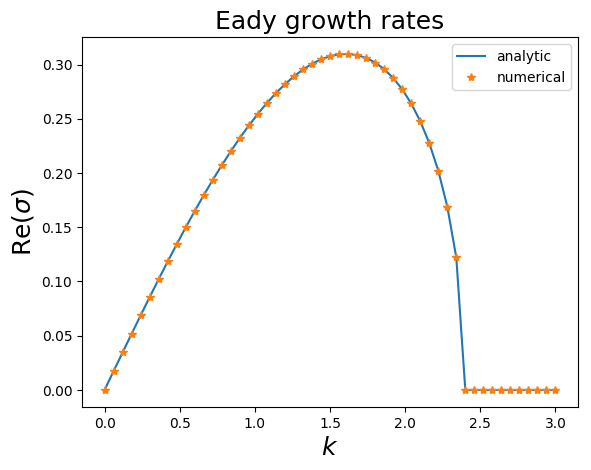Now let's find the eigenvectors of $\mathbb{A}_{\mathbb{M}}$, $\mathbb{A}_{\mathbb{M}}^\dagger$, $\mathbb{A}_{\mathbb{M}}+\mathbb{A}_{\mathbb{M}}^\dagger$, and $e^{\mathbb{A}^\dagger t_*}e^{\mathbb{A} t_*}$ for some finite time $t_*$. Remember that to plot we need to return back to streamfunction variables by acting with $\mathbb{M}^{-1/2}$.

In :
function eigenanalysis(A)
S, U = eigen(A);
mostunstable = findall(real(S) .== maximum(real(S)))
return S[mostunstable], U[:, mostunstable]
end

function find_phi0s(k, tstar)
# finds the eigenvector of A, A^\dagger, A+A^\dagger,
# and e^{A^dagger t}e^{A t} for t=tstar

A, M, AM, Msqrt, invMsqrt = create_operators(k)
maxgrowthrate = maximum(real(eigvals(A)))

s1, u1 = eigenanalysis(AM);
s1inst, w1 = eigenanalysis(AM + Matrix(adjoint(AM)));
return s1, u1, s1adj, v1, s1inst, w1, s1opt, y1
end;

In :
function plot_phi0s(;k=1.6, tstar=1)
# plots the eigenvector of A, A^\dagger, A+A^\dagger,
# and e^{A^dagger t}e^{A t} for t=tstar

A, M, AM, Msqrt, invMsqrt = create_operators(k)
s1, u1, s1adj, v1, s1inst, w1, s1opt, y1 = find_phi0s(k, tstar)

nx = 100
x = range(0, stop=2π, length=nx)
eikx = Matrix(transpose(exp.(im*k*x)))

# go back to 'normal' coordinates by multiplying with invMsqrt
u1real = real.((invMsqrt*u1)*eikx)
v1real = real.((invMsqrt*v1)*eikx)
w1real = real.((invMsqrt*w1)*eikx)
y1real = real.((invMsqrt*y1)*eikx)

fig, axs = subplots(ncols=2, nrows=2, figsize=(6.5, 6.5))
sca(axs); contour(x, z, u1real)
title(L"eigvector of $A$", fontsize=14)
ylabel(L"$z$", fontsize=14)
sca(axs); contour(x, z, v1real)
title(L"eigvector of $A^\dagger$", fontsize=14)
xlabel(L"$x$", fontsize=14); ylabel(L"$z$", fontsize=14)
sca(axs); contour(x, z, w1real)
title(L"eigvector of $A+A^\dagger$", fontsize=14)
sca(axs); contour(x, z, y1real)
title("eigvector of \$e^{A^\\dagger t_*}e^{A t_*}\$, \$t_*=$tstar\$", fontsize=14) xlabel(L"$x$", fontsize=14) end;  In : plot_phi0s(k=1.6, tstar=5);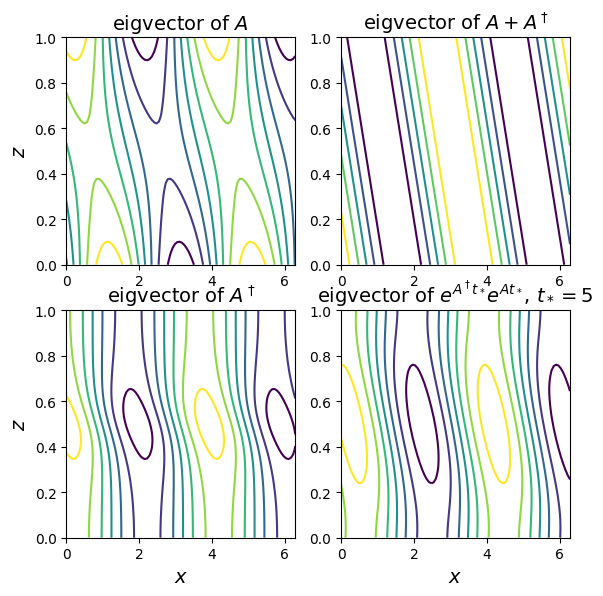Now let's see how the perturbation energy evolves if we use each of the previous eigenvectors as an initial condition. In : function compute_energy(phi0, tfinal, AM) t = range(0, stop=tfinal, length=101) energy = zeros(length(t)) dt = t-t expAMdt = exp(AM*dt) phit = phi0/sqrt(dot(phi0, phi0)) for j in 1:length(t) energy[j] = dot(phit, phit) phit .= expAMdt*phit end return t, energy end;  In : k=1.6 A, M, AM, Msqrt, invMsqrt = create_operators(k) tstar, tfinal = 5, 10 s1, u1, s1adj, v1, s1inst, w1, s1opt, y1 = find_phi0s(k, tstar) figure(figsize=(6, 2.8)) t, energy = compute_energy(u1, tfinal, AM) semilogy(t, energy , label="eigenvector") t, energy = compute_energy(v1, tfinal, AM) semilogy(t, energy , label="adjoint") t, energy = compute_energy(w1, tfinal, AM) semilogy(t, energy , label="instantaneous") t, energy = compute_energy(y1, tfinal, AM) semilogy(t, energy , label="optimal \$t_*= $tstar\$")
semilogy(t, exp.(2*real(s1)*t), "--k")
xlabel(L"$t$", fontsize=16); ylabel(L"$E(t)$", fontsize=16)
legend();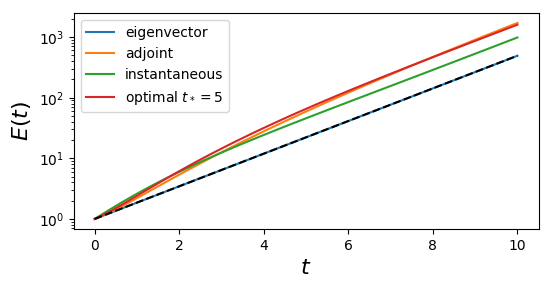We see that the eigenmode grows the least of all!

What's even more interesting is to repeat all these but for a zonal wavenumber $k$ for which there is not any unstable eigenmode of $\mathbb{A}$. For example, let's do it for $k=2.6$.

In :
plot_phi0s(k=2.6, tstar=5);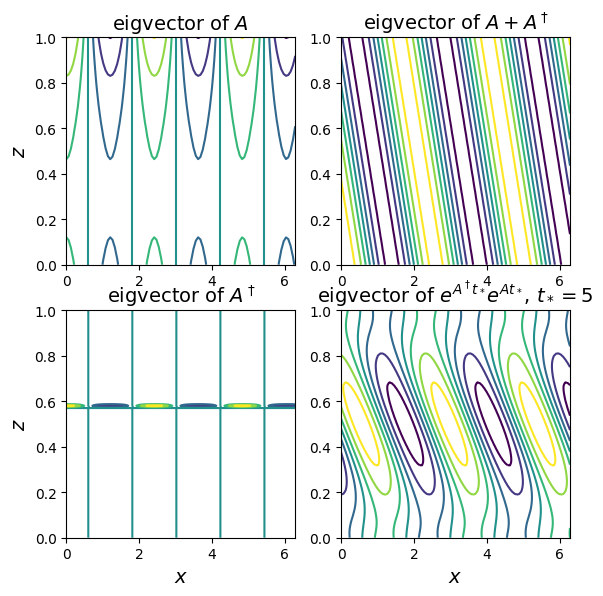In :
k=2.6
A, M, AM, Msqrt, invMsqrt = create_operators(k)

tstar, tfinal = 5, 10
s1, u1, s1adj, v1, s1inst, w1, s1opt, y1 = find_phi0s(k, tstar)

figure(figsize=(6, 2.8))
t, energy = compute_energy(u1, tfinal, AM); semilogy(t, energy , label="eigenvector")
t, energy = compute_energy(v1, tfinal, AM); semilogy(t, energy , label="adjoint")
t, energy = compute_energy(w1, tfinal, AM); semilogy(t, energy , label="instantaneous")
t, energy = compute_energy(y1, tfinal, AM); semilogy(t, energy , label="optimal \$t_*=10\$")
xlabel(L"$t$", fontsize=16); ylabel(L"$E(t)$", fontsize=16)
legend();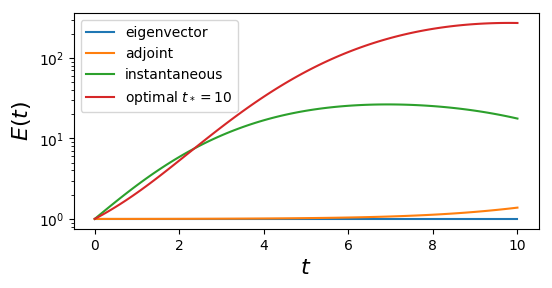What if we plot for larger times?

In :
k=2.6
A, M, AM, Msqrt, invMsqrt = create_operators(k)

tstar, tfinal = 5, 100
s1, u1, s1adj, v1, s1inst, w1, s1opt, y1 = find_phi0s(k, tstar)

figure(figsize=(6, 2.8))
t, energy = compute_energy(u1, tfinal, AM); semilogy(t, energy , label="eigenvector")
t, energy = compute_energy(v1, tfinal, AM); semilogy(t, energy , label="adjoint")
t, energy = compute_energy(w1, tfinal, AM); semilogy(t, energy , label="instantaneous")
t, energy = compute_energy(y1, tfinal, AM); semilogy(t, energy , label="optimal \$t_*=10\$")
xlabel(L"$t$", fontsize=16); ylabel(L"$E(t)$", fontsize=16)
legend();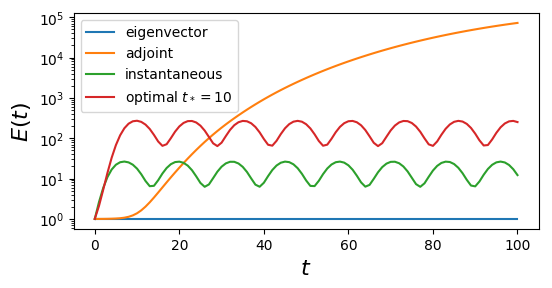The adjoint eigenmode grows in energy up to $10^5$ times...

Exercise: Try looking for what happens for even longer times.

So it's apparent that for non-normal operators eigenanalysis does not tell us much for how the perturbations will actually evolve.

As a last example let's consider the following. From the eigenanalysis of the problem we see that there is a high-wavenumber cutoff of the Eady instability. That is for $k_1<k_{\mathrm{crit}}\approx2.4$ there is instability while for $k_2>k_{\mathrm{crit}}$ all eigenvalues of $\mathbb{A}$ have negative real part.

Let's pick two wavenumbers close to the $k_{\mathrm{crit}}$ and investigate what's the maximum perturbation growth that we can get.

In :
tstar, tfinal = 10, 50
k1 = 2.38 # this is <k_crit
A1, M1, AM1, M1sqrt, invM1sqrt = create_operators(k1)
s1, u1, s1adj, v1, s1inst, w1, s1opt, y1 = find_phi0s(k1, tstar)
t1, energy1 = compute_energy(y1, tfinal, AM1)
k2 = 2.42 # this is >k_crit
A2, M2, AM2, M2sqrt, invM2sqrt = create_operators(k2)
s2, u2, s2adj, v2, s2inst, w2, s2opt, y2 = find_phi0s(k2, tstar);
t2, energy2 = compute_energy(y2, tfinal, AM2)
gr1, gr2 = round(real(s1), digits=3), round(real(s2), digits=3)

figure(figsize=(6, 2.8))
semilogy(t1, energy1, label="\$k_1=$k1 \$, \$\\sigma_1 = $gr1\$")
semilogy(t2, energy2, label="\$k_2=$k2 \$, \$\\sigma_2 = $gr2\$")
semilogy(t1, 2e3*exp.(2*real(s1)*t1), "--k", linewidth=0.8, label="\$\\propto e^{2\\mathrm{Re}(\\sigma_1)t}\$")
semilogy(t1, 1e3*exp.(2*real(s2)*t1), "-.k", linewidth=0.8, label="\$\\propto e^{2\\mathrm{Re}(\\sigma_2)t}\$")
xlabel(L"$t$", fontsize=16); ylabel(L"$E(t)/E(0)$", fontsize=16); legend();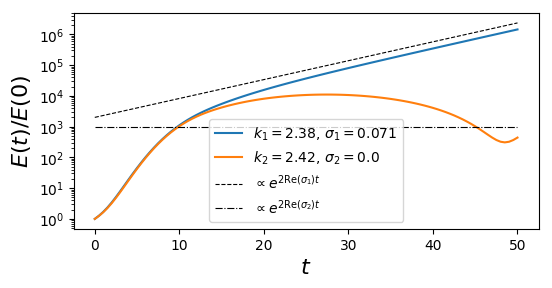We see that for both wavenumbers if we start with the eigenvector of $\mathbb{A}_{\mathbb{M}}^\dagger$ as initial condition the energy evolution is same for up to $t\approx 10$. Beyond that point the energy evolution goes as $e^{2\mathrm{Re}(\sigma_1)t}$ for $k_1<k_{\mathrm{crit}}$, while it oscillates about a constant value for $k_2$.

But regardless of whether there was an instability or not, both wavenumbers showed perturbation energy growth of about $10^3$ before they diverge.

This brings us back to what we were discussing in the first lectures: Eigenanalysis or modal stability only tells you what will happen at $t\to\infty$. Indeed, at $t\to\infty$ the energy for $k_1$ and $k_2$ is very different. But that's not the case at all for intermediate times (in this example for up to $t\approx 10$).

The following code evoles an initial condition and plots snapshots of the streamfunction and the energy evolution.

In [ ]:
k, tstar = 2.6, 5
A, M, AM, Msqrt, invMsqrt = create_operators(k)
s1, u1, s1adj, v1, s1inst, w1, s1opt, y1 = find_phi0s(k, tstar)

phi0 = y1
phit = phi0 / sqrt(dot(phi0, phi0))

tfinal = 10
t = range(0, stop=tfinal, length = 51)
dt = t-t
energy = zeros(length(t))
expAMdt = exp(AM*dt)

nx = 100
x = range(0, stop=2π, length=nx)
eikx = Matrix(transpose(exp.(im*k*x)))

fig, axs = subplots(ncols=2, nrows=1, figsize=(12, 2.8))

for j in 1:length(t)
energy[j] = dot(phit, phit)
phit = expAMdt*phit

phitreal = real.((invMsqrt*phit)*eikx)

sca(axs); cla();
contour(x, z, phitreal)
xlabel(L"$x$", fontsize=14)
ylabel(L"$z$", fontsize=14)
sca(axs); cla();
semilogy(t[1:j], energy[1:j])
xlabel(L"$t$", fontsize=14)
ylabel(L"$E(t)$", fontsize=14)
sleep(0.01)
IJulia.clear_output(true)
display(fig)
end

• Vallis (2017). Atmospheric and oceanic fluid dynamics. Cambridge University Press.
• Farrell (1984). Modal and non-modal baroclinic waves, J. Atmos. Sci., 41 (4), 668-673.